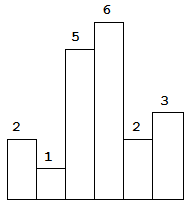# Leetcode: Largest Rectangle in Histogram

Largest Rectangle in HistogramSimilar Problems:

Given n non-negative integers representing the histogram’s bar height where the width of each bar is 1, find the area of largest rectangle in the histogram.Above is a histogram where width of each bar is 1, given height = [2,1,5,6,2,3].

The largest rectangle is shown in the shaded area, which has area = 10 unit.

For example,
Given heights = [2,1,5,6,2,3],
return 10.

Github: code.dennyzhang.com

Credits To: leetcode.com

Leave me comments, if you have better ways to solve.

```## Blog link: https://code.dennyzhang.com/largest-rectangle-in-histogram
## Basic Ideas: Monotone stack
##              For each element, find the next non-greater value
##     Key Questions: How to get the left?
##
## Complexity:
class Solution(object):
def largestRectangleArea(self, heights):
"""
:type heights: List[int]
:rtype: int
"""
length = len(heights)
next_smallers = [-1] * length
stack = []
max_width = 0
# pad with fake items for the end
for i in xrange(length+1):
current = heights[i] if i != length else -1
# When heights[i] is not greater than the stack top, it's the target of stack top
while len(stack) != 0 and  current <= heights[stack[-1]]:
k = stack.pop()
h = heights[k]
left = -1 if len(stack) == 0 else stack[-1]
left = left + 1
right = i
max_width = max(max_width, h*(right-left))
stack.append(i)
return max_width

# s = Solution()
# print s.largestRectangleArea([2, 1, 2]) # 3
```

Share It, If You Like It.